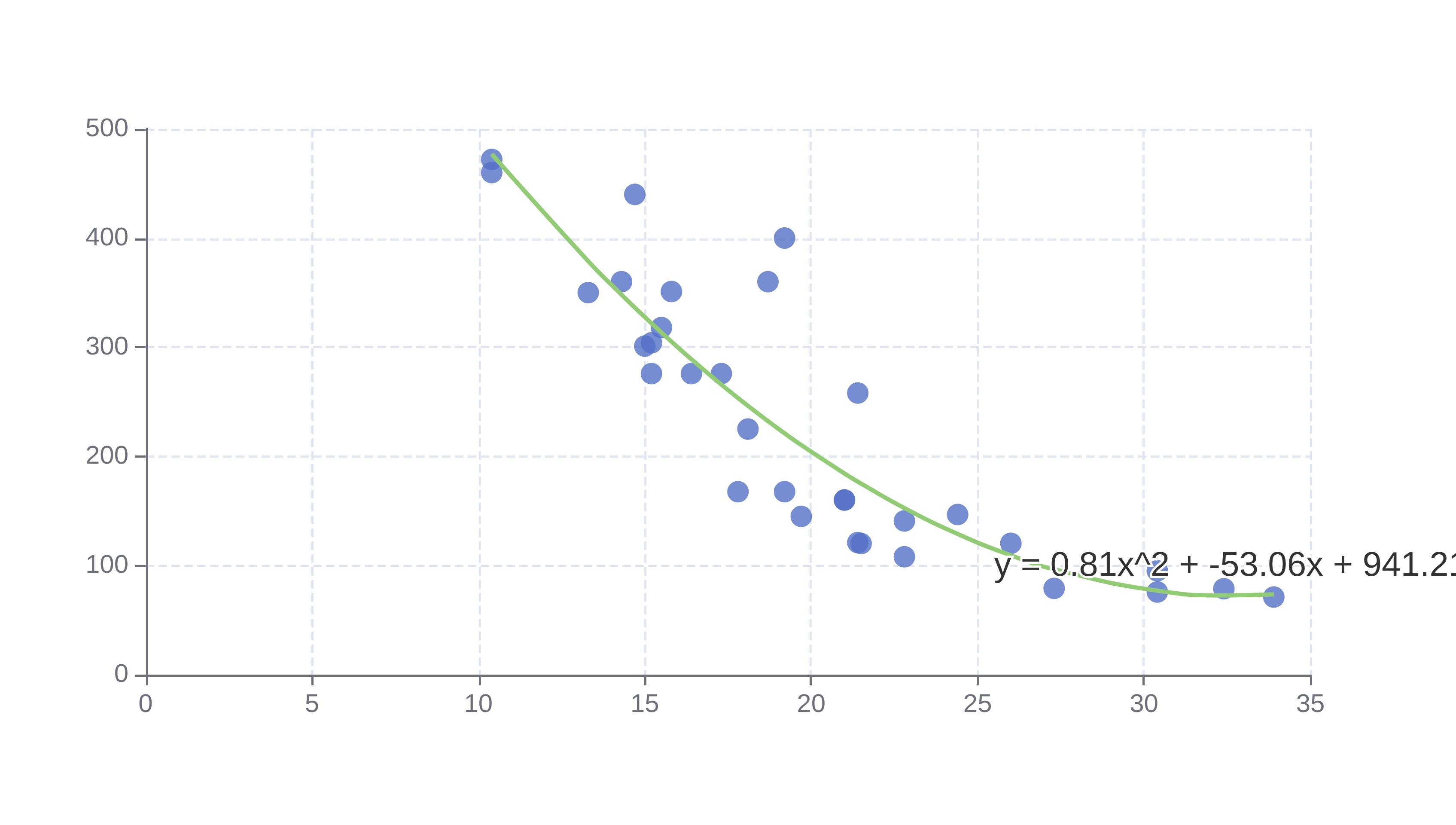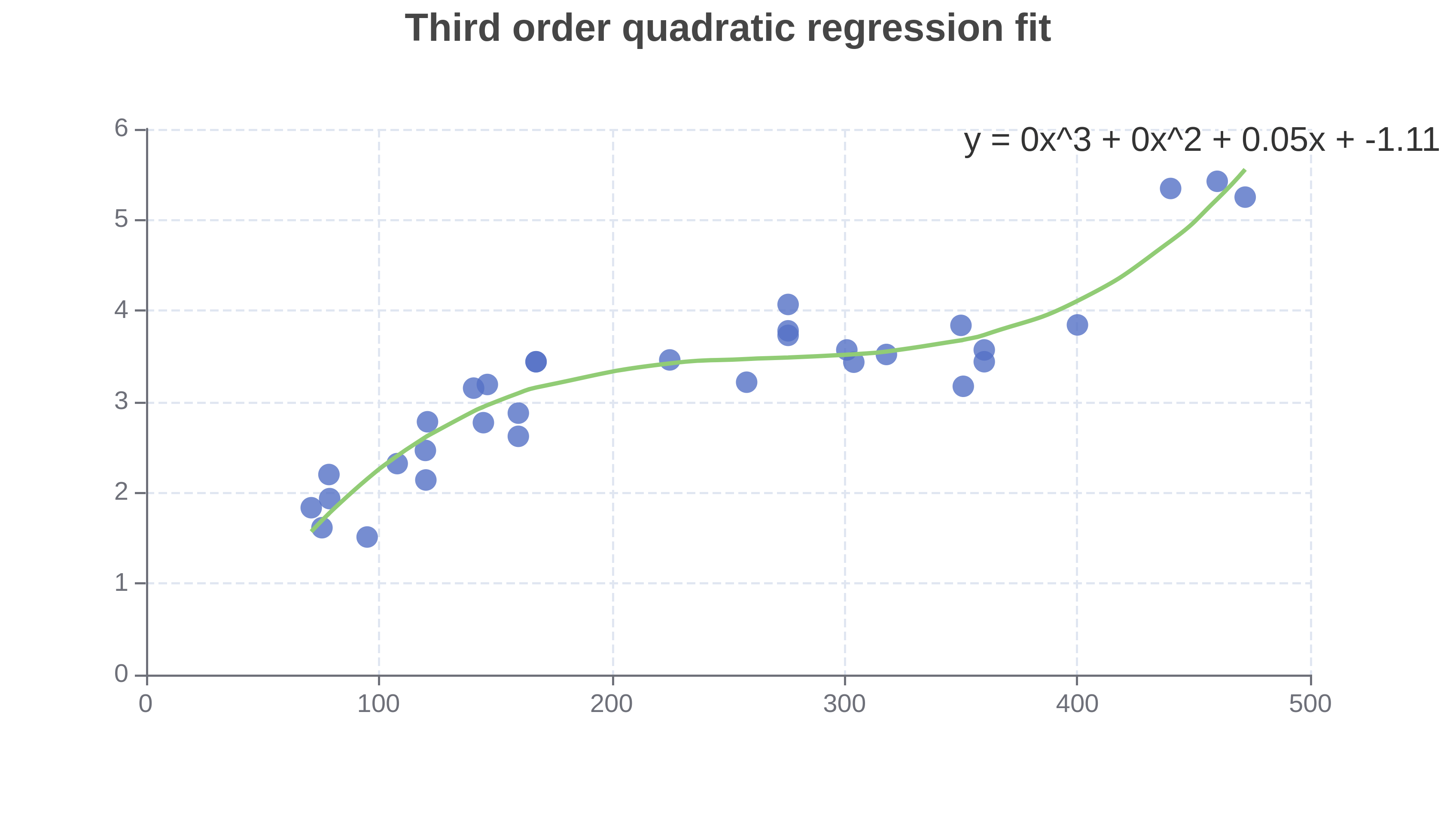# Polynomial Regression Calculator

Or input your data as csv

Sharing helps us build more free tools

Polynomial Regression models the relationship between the input variables with an nth-degree polynomial model. This means it can fit non linear relationships between the input variables.

The polynomial regression fit below shows the fit between miles per gallon fuel consumption and engine displacement in the mt cars data set.The independent variable is plotted on the x axis and the dependent variable is plotted on the y axis.

Polynomial regression is a type of linear regression analysis. Cubic regression is a special case of polynomial regression where a cubic function is fit. Quadratic regression is a special case of polynomial regression where a second-order function is fit.

The linear regression calculator only fits a straight line to input data. The exponential regression calculator models the relationship between the input data as an exponential function

Polynomial regression is a useful tool when the relationship between the input data can be modeled accurately with a polynomial function. If the relationship can be better modeled with a straight line, then the linear regression calculator would be a more useful tool.

If it looks like there is an exponential relationship between the input data then the exponential regression calculator is a better tool to use.

## When To Use Polynomial Regression

Polynomial regression is a useful tool when the relationship between the input data can be modeled accurately with a polynomial function. If the relationship can be better modeled with a straight line, then the linear regression calculator would be a more useful tool. If it looks like there is an exponential relationship between the input data then the exponential regression calculator is a better tool to use.

## How To Perform Polynomial Regression

Click the dataset input at the top of the page. Or drag and drop your dataset into the input box.

2. ### View the polynomial regression fit

A polynomial regression fit will appear on the screen

3. ### Adjust the polynomial order

Change the order of the fit. If it is underfitting you can increase the polynomial order. If it is overfitting, then you can reduce the order to make it closer to a linear fit.

### How to Find the Best Fit Third Degree Polynomial: y = ax^3 + bx^2 + cx + d

The cubic regression equation looks a lot like the second-order regression equationThe only thing that changed is we added an ax^3 term at the front.

This extra term means that our regression line can be more curved to fit higher-order relationships. The third-order regression line in the chart below curves up and down.

The chart below shows a third-order fit calculated with the polynomial regression calculator.We can keep adding terms to the front to get higher-order polynomial regression equations.